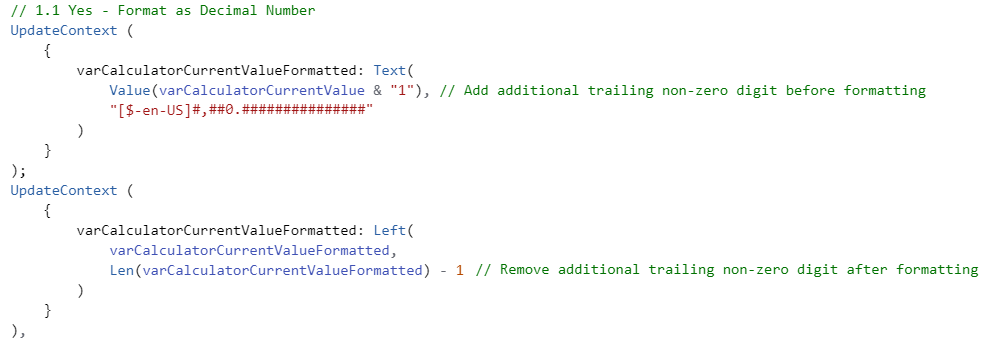# How to format numbers stored and displayed as text in a Canvas Power App

### Colin Maitland, 17 December 2019

In this article, I demonstrate how to format the display of a number in a Canvas Power App as it is being entered by the user. This demonstration uses the example of a custom calculator created using various buttons, labels and formulas and which allows the user to enter up to 12 numeric digits as part of each step of a calculation.

In this scenario it is desired that every digit and any decimal point entered by the user is displayed in a format that adds commas; only displays a decimal point if one has been entered; always displays any decimal point even when there are no digits following the decimal point; and always displays all trailing zeros after the decimal point even when there are no trailing non-zero digits after these.

The top number in the calculator display is the result of the most recently completed calculation. This number is stored as a number and is formatted using standard numeric format; e.g, “0”, “0.”, and “0.0” should all be displayed as “0”.

The bottom number in the calculator display is the number currently being entered by the user. The bottom number is stored as text and is formatted according to the previously described rules; e.g. “0”, “0.”, and “0.0” should all be displayed as such with the removal of any trailing decimal point or “0”.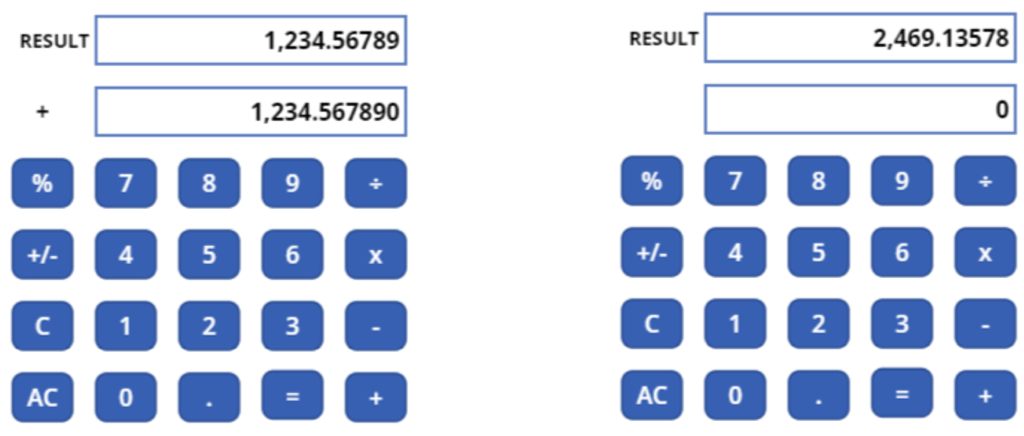The following formula is used to format the display of the top number.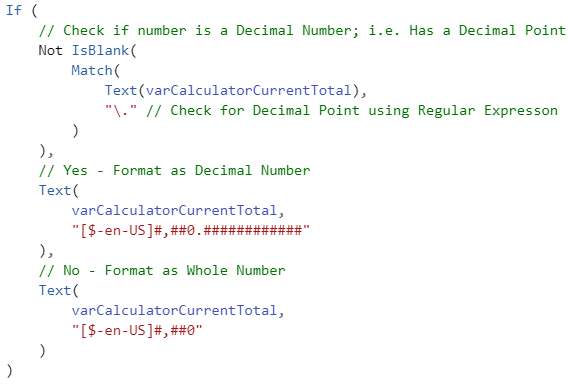The following table shows examples of the desired format for the bottom number being entered by the user. The unformatted value is what is entered by the user and the formatted value is what should be displayed.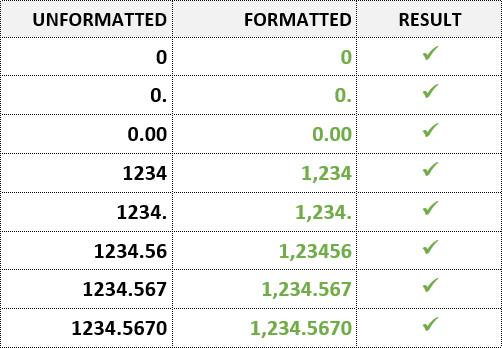The Text function is used to format these numbers. Each of the following five progressive examples demonstrate Text function format parameters that do not produce these desired results in all cases. However, the final and sixth example demonstrates a working solution. You will notice that in some of the examples the formatted number is blank and in others, the last digit of the formatted number is rounded up. This behaviour is standard. Refer to https://docs.microsoft.com/en-us/powerapps/maker/canvas-apps/functions/function-text for detailed information on the Text function.

In these examples, the unformatted number entered by the user is stored as a text value in a Context Variable named “varCalculatorCurrentValue”. The number is stored as text so that all characters entered by the user are preserved for display back to the user; e.g. for decimal numbers, the decimal point, when there are no following non-zero digits and all following zero’s when there are no following non-zero digits.

### Example 1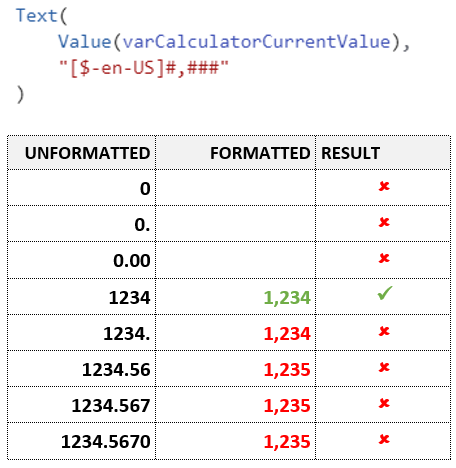### Example 2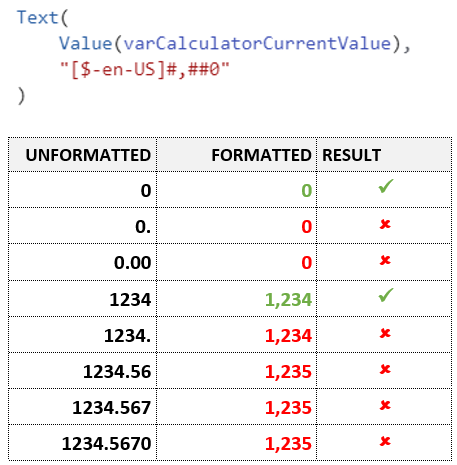### Example 3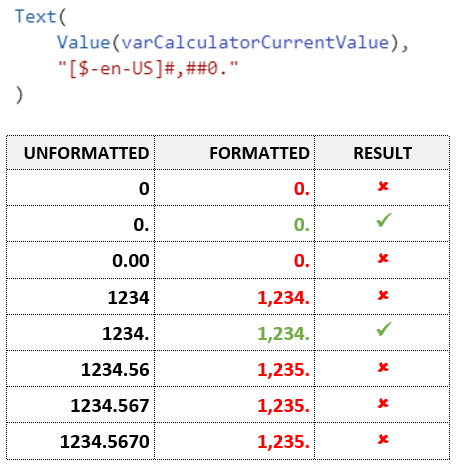### Example 4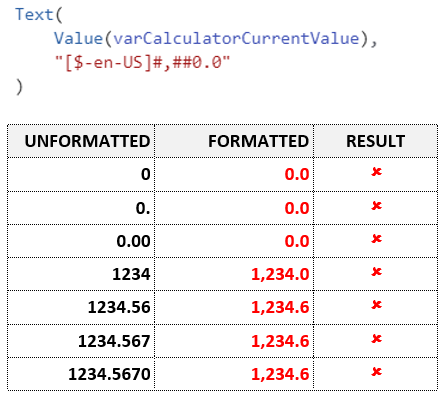### Example 5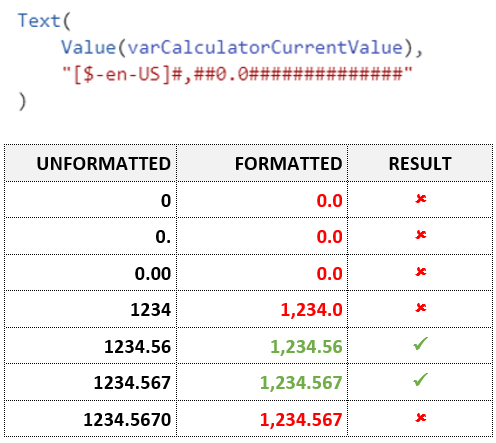### Example 6

None of the previous examples produces the desired result in all cases. However, this final example does as long as the number of decimal digits entered by the user is less than 15.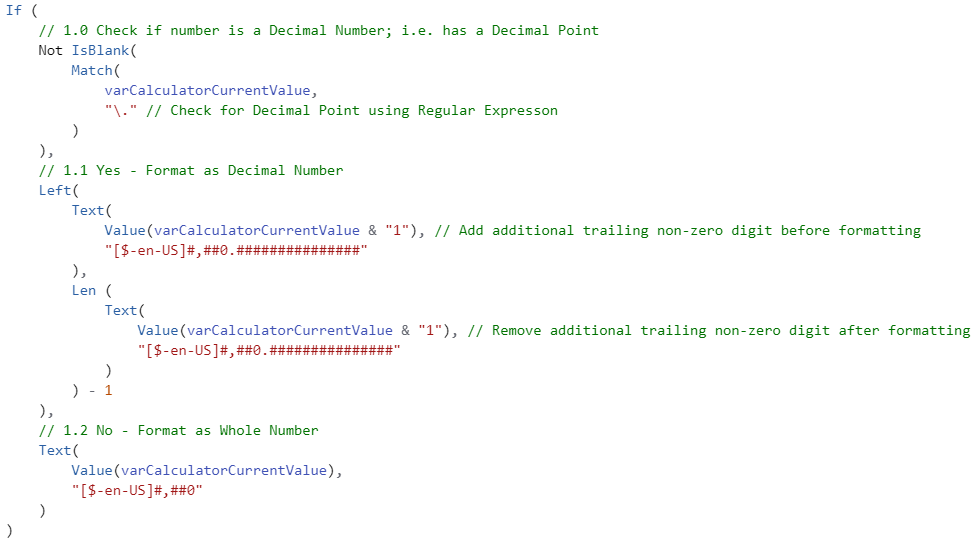This approach works because:

Firstly, there are different branches for formatting numbers that contain a decimal point and numbers that don’t.

Secondly, in the branch that formats numbers that contain a decimal point, there are steps to append a non-zero digit as the last digit before any formatting is applied and then remove it again after the formatting is applied. This approach ensures that all trailing zeros entered by the user, if any, are retained when the formatting is applied. However, to ensure that no 0’s are displayed after the decimal point when none have been entered by the user, the format is “0.#” rather than “0.0” around the decimal point. The format allows for up to fifteen digits to be displayed after the decimal point. The functionality associated with the custom calculator used in this example does not permit the user to enter more than 12 numeric digits in total.

Finally, you will notice that the branch that formats decimal numbers use the Text function with the addition of a non-zero last digit and formatting twice! The second occurrence is to obtain the length of the formatted number so that the extra digit can be removed again using the Left function. Because this formula is associated with the Text property of the label control in which the formatted number is displayed, it is not possible to optimise this step by assigning the formatted number to a context variable and then getting the length from the context variable for use by the Left function as shown below. This is because the UpdateContext function is a behaviour function, but the Text property of the control is a non-behaviour property. The two cannot be used together. In addition, there is no reduction in the formula line count.